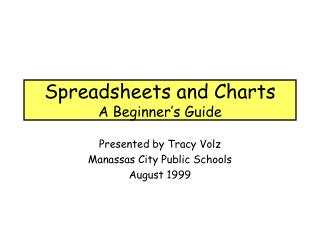DownloadDownload PresentationSpreadsheets and Charts A Beginner’s Guide

# Spreadsheets and Charts A Beginner’s Guide

Télécharger la présentation## Spreadsheets and Charts A Beginner’s Guide

- - - - - - - - - - - - - - - - - - - - - - - - - - - E N D - - - - - - - - - - - - - - - - - - - - - - - - - - -
##### Presentation Transcript

1. Spreadsheets and ChartsA Beginner’s Guide Presented by Tracy Volz Manassas City Public Schools August 1999

2. What is a Spreadsheet?A spreadsheet ... • is an electronic version of a ledger • consists of a grid from columns and rows • is a computation tool • can accurately compute mathematical formulas • helps organize, display, and manage information • has the ability to create charts and graphs • can be used to create a tables

3. A spreadsheet is ... the electronic version of a ledger. It is a grid made from columns and rows. The nice thing about using a spreadsheet is that you can experiment with numbers without having to RE-DO all of the calculations!

4. Basics of a Spreadsheet • Spreadsheets are made up of • columns • rows • cells • Each cell contains a type of data • text • number data • formulas

5.  COLUMNS…  COLUMNS go vertically - up and down. They are defined by Letters which go across the top of the grid.

6.  ROWS…  ROWS go horizontally - left to right Rows are defined by Numbers which go down the left side of the grid.

7. CELL... The CELL is the space where the column and the row intersect. B6 The CELL is named according to its Column letter and Row number. It is where the information is stored.

8.  Types of Data  Cellscan contain three types of data. LABEL - anything that is just text ex. Title or Items or Cost CONSTANT - any number ex. 8 or 3.14 or -59.6 FORMULA - math equation ex. =8*5 or =6+8/4

9.  LABELS  LABELS help us identify what the numbers stand for… they help us understand the spreadsheet. LABELS are text entries - they have no numerical value.

10.  CONSTANTS  Constants are used to enter FIXED number data. A constant can be any kind of specific number: currency, percent, decimal ...

11. # Working with Constants # When you are working with constants or values, you must use the appropriate symbols. + is the symbol foraddition -is the symbol forsubtraction *is the symbol for multiplication /is the symbol for division

12.  FORMULAS  Formulas are equations that performs calculations. All formulasMUST BEGIN with an equal sign (=). A formula will be displayed on the screen as a number, but the entry bar will show the formula.

13.  A Few Formulas  Suppose you had a spreadsheet showing test grades… you might use the following formulas. To Find Use Total Scores =sum( __: __ ) High Score =max( __:__ ) Low Score =min( __:__ ) Average Score =average( __:__ ) Total # of Scores =count( __:__ ) (It will not count text entries or blank entries!) (

14.  IF Function  Example of IF=IF (A3>70, “Pass”, “Fail”) Compares Is Cell A3 greater than 70? Answer If Cell A3 is greater than 70, then it will print Pass. If Cell A3 is less than 70, then it will print Fail.

15.  Charts or Graphing  You can use the Chart Wizard to help you make a chart or graph. There are 16 types of charts you can choose from. The type of chart you pick usually depends on the data you are using! For more information on Charts go to: http://help.unc.edu/cgi-bin/getdocs?docnumber=dwe28

16.  Don’t Forget!!!  “Like ANY tool, a spreadsheet should be used to aide in producing a polished product and NOT become the product.” - Teacher Created Materials

17.  Let’s Try It Out!!!!  • Let’s Go Shopping!!! • Create a Quiz • Questions & Answers • Check It Out!!! Ideas taken from http://www.usd.edu/trio/excel/1.html

18. 3-2-1 Summary 3 Write 3 New Things You Learned Today 2 Write 2 Things You Would Like to Know More About 1 Write 1 Instructional Thing You Will Share with a Teacher